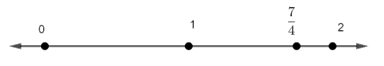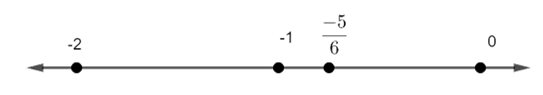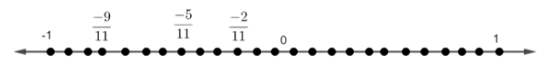Courses
Courses for Kids
Free study material
Offline Centres
More

# NCERT Solutions for Class 8 Maths Chapter 1 - ExerciseLast updated date: 30th Nov 2023
Total views: 529.5k
Views today: 8.29k

## NCERT Solutions for Class 8 Maths Chapter 1 Rational Numbers (EX 1.2) Exercise 1.2

NCERT Solutions for Class 8 Math Chapter 1 are provided by Vedantu. The very first chapter in the Class 8 Maths syllabus, ‘Rational Numbers’, and the exercise 1.2 Class 8 is a critical and important part of the Maths syllabus. So, if you are in search of assistance with the solutions to the exercise problems in the NCERT textbook, then there cannot be a better alternative than Vedantu’s Class 8 Math Chapter 1. You can download this in PDF format prepared by our experts based on the CBSE guidelines. Vedantu is a platform that provides free NCERT Solution and other study materials for students. Science students who are looking for NCERT Solutions for Class 8 Science will also find the solutions curated by our Master Teachers really helpful.

## Important Properties under NCERT Solutions for Class 8 Math Chapter 1 Rational Numbers (EX 1.2) Exercise 1.2

The first chapter of the class 8 math syllabus is on Rational Numbers. It is a significant chapter in math that is covered in class 8.  NCERT Class 8 Math Chapter 1 Rational Numbers has 6 major properties that are dealt with in the solutions. The following is a list of the 6 important properties that come under the chapter Rational Numbers.

• The Closure Property.

• The Commutative Property.

• The Associative Property.

• The Distributive Property.

• The Identity Property.

• The Inverse Property.

### Importance of Rational Numbers

Rational numbers help describe various measures and quantities that integers alone cannot describe fully. Rational numbers are mostly used to measure quantities like mass, length, time, and so on.

Access NCERT Solutions for class 8 Math  Chapter 1 – Rational Numbers

Exercise 1.2

1. Represent these numbers on the number line.

1. $\dfrac{7}{4}$

Ans: Since, $\dfrac{7}{4}$ is approximately equal to $1.75$.

Therefore, $\dfrac{7}{4}$ can be represented on the number line as follows:1. $-\dfrac{5}{6}$

Ans: Since, $-\dfrac{5}{6}$ is approximately equal to $-0.833$.

Thus, $-\dfrac{5}{6}$ can be represented on the number line as follows:2.Represent $\text{-}\dfrac{\text{2}}{\text{11}}\text{,-}\dfrac{\text{5}}{\text{11}}\text{,-}\dfrac{\text{9}}{\text{11}}$ on the number line.

Ans: We can divide the interval between $\text{0}$ and $\text{-1}$ in $\text{11}$ parts to get.

$\text{-}\dfrac{\text{2}}{\text{11}}\text{,-}\dfrac{\text{5}}{\text{11}}\text{,-}\dfrac{\text{9}}{\text{11}}$ on number line.

Thus, $\text{-}\dfrac{\text{2}}{\text{11}}\text{,-}\dfrac{\text{5}}{\text{11}}\text{,-}\dfrac{\text{9}}{\text{11}}$ can be represented on the number line as follows:3. Write five rational numbers which are smaller than $\text{2}$.

Ans: Since, we have to write five rational numbers which are less than $\text{2}$ .

Therefore, we can multiply and divide $\text{2}$ by $7$.

Now, $\text{2}$ becomes $\dfrac{\text{14}}{\text{7}}$.

Thus, five rational numbers which are smaller than $\text{2}$ are given as:

$\dfrac{\text{13}}{\text{7}}\text{,}\dfrac{\text{12}}{\text{7}}\text{,}\dfrac{\text{11}}{\text{7}}\text{,}\dfrac{\text{10}}{\text{7}}\text{,}\dfrac{\text{9}}{\text{7}}$.

4. Find ten rational numbers between $\dfrac{\text{-2}}{\text{5}}$ and $\dfrac{\text{1}}{\text{2}}$.

Ans: We can make denominator of  $\dfrac{\text{-2}}{\text{5}}$ and $\dfrac{\text{1}}{\text{2}}$ same.

Therefore, multiplying and dividing $\dfrac{\text{-2}}{\text{5}}$ by $\text{4}$ and $\dfrac{\text{1}}{\text{2}}$ by $\text{10}$.

Thus, now $\dfrac{\text{-2}}{\text{5}}$ becomes $\dfrac{\text{-8}}{\text{20}}$  and  $\dfrac{\text{1}}{\text{2}}$ becomes $\dfrac{\text{10}}{\text{20}}$ .

Hence, ten rational numbers between $\dfrac{\text{-2}}{\text{5}}$ and $\dfrac{\text{1}}{\text{2}}$ are:

$\text{-}\dfrac{\text{7}}{\text{20}}\text{,-}\dfrac{\text{6}}{\text{20}}\text{,-}\dfrac{\text{5}}{\text{20}}\text{,-}\dfrac{\text{4}}{\text{20}}\text{,-}\dfrac{\text{3}}{\text{20}}\text{,-}\dfrac{\text{2}}{\text{20}}\text{,-}\dfrac{\text{1}}{\text{20}}\text{,0,}\dfrac{\text{1}}{\text{20}}\text{,}\dfrac{\text{2}}{\text{20}}$.

5. Find five rational numbers between-

1. $\dfrac{\text{2}}{\text{3}}$ and $\dfrac{\text{4}}{\text{5}}$

Ans: We can make denominator of  $\dfrac{\text{2}}{\text{3}}$ and $\dfrac{\text{4}}{\text{5}}$ same.

Therefore, multiplying and dividing $\dfrac{\text{2}}{\text{3}}$ by $\text{15}$ and $\dfrac{\text{4}}{\text{5}}$ by $9$.

Thus, now $\dfrac{\text{2}}{\text{3}}$ becomes $\dfrac{\text{30}}{\text{45}}$  and  $\dfrac{\text{4}}{\text{5}}$ becomes $\dfrac{\text{36}}{\text{45}}$ .

Hence, ten rational numbers between $\dfrac{\text{2}}{\text{3}}$ and $\dfrac{\text{4}}{\text{5}}$ are:

$\dfrac{\text{31}}{\text{45}}\text{,}\dfrac{\text{32}}{\text{45}}\text{,}\dfrac{\text{33}}{\text{45}}\text{,}\dfrac{\text{34}}{\text{45}}\text{,}\dfrac{\text{35}}{\text{45}}$.

1. $\dfrac{\text{-3}}{\text{2}}$ and $\dfrac{\text{5}}{\text{3}}$.

Ans: We can make denominator of  $\text{-}\dfrac{\text{3}}{\text{2}}$ and $\dfrac{\text{5}}{\text{3}}$ same.

Therefore, multiplying and dividing $\text{-}\dfrac{\text{3}}{\text{2}}$ by $3$ and $\dfrac{\text{5}}{\text{3}}$ by $2$.

Thus, now $\text{-}\dfrac{\text{3}}{\text{2}}$ becomes $\text{-}\dfrac{\text{9}}{\text{6}}$  and  $\dfrac{\text{5}}{\text{3}}$ becomes $\dfrac{\text{10}}{\text{6}}$ .

Hence, ten rational numbers between $\text{-}\dfrac{\text{3}}{\text{2}}$ and $\dfrac{\text{5}}{\text{3}}$ are:

$\text{-}\dfrac{\text{8}}{\text{6}}\text{,-}\dfrac{\text{7}}{\text{6}}\text{,-1,-}\dfrac{\text{5}}{\text{6}}\text{,-}\dfrac{\text{4}}{\text{6}}$.

1. $\dfrac{\text{1}}{\text{4}}$ and $\dfrac{\text{1}}{\text{2}}$

Ans: We can make denominator of  $\dfrac{\text{1}}{\text{4}}$ and $\dfrac{\text{1}}{\text{2}}$ same.

Therefore, multiplying and dividing $\dfrac{\text{1}}{\text{4}}$ by $8$ and $\dfrac{\text{1}}{\text{2}}$ by $16$.

Thus, now $\dfrac{\text{1}}{\text{4}}$ becomes $\dfrac{\text{8}}{\text{32}}$  and  $\dfrac{\text{1}}{\text{2}}$ becomes $\dfrac{\text{16}}{\text{32}}$ .

Hence, ten rational numbers between $\dfrac{\text{1}}{\text{4}}$ and $\dfrac{\text{1}}{\text{2}}$ are:

$\dfrac{\text{9}}{\text{32}}\text{,}\dfrac{\text{10}}{\text{32}}\text{,}\dfrac{\text{11}}{\text{32}}\text{,}\dfrac{\text{12}}{\text{32}}\text{,}\dfrac{\text{13}}{\text{32}}$.

6. Write five rational numbers greater than $\text{-2}$.

Ans:

Since, we have to write five rational numbers which are greater than $\text{-2}$.

Therefore, we can multiply and divide $\text{-2}$ by $7$.

Now, $\text{-2}$ becomes $\text{-}\dfrac{\text{14}}{\text{7}}$.

Thus, five rational numbers greater than $\text{-2}$ are given as:

$\text{-}\dfrac{\text{13}}{\text{7}}\text{,-}\dfrac{\text{12}}{\text{7}}\text{,-}\dfrac{\text{11}}{\text{7}}\text{,-}\dfrac{\text{10}}{\text{7}}\text{,-}\dfrac{\text{9}}{\text{7}}$.

7. Find ten rational numbers between $\dfrac{\text{3}}{\text{5}}$ and $\dfrac{\text{3}}{\text{4}}$.

Ans: We can make denominator of  $\dfrac{\text{3}}{\text{5}}$ and $\dfrac{\text{3}}{\text{4}}$ same.

Therefore, multiplying and dividing $\dfrac{\text{3}}{\text{5}}$ by $16$ and $\dfrac{\text{3}}{\text{4}}$ by $20$.

Thus, now $\dfrac{\text{3}}{\text{5}}$ becomes $\dfrac{\text{48}}{\text{80}}$  and  $\dfrac{\text{3}}{\text{4}}$ becomes $\dfrac{\text{60}}{\text{80}}$ .

Hence, ten rational numbers between $\dfrac{\text{3}}{\text{5}}$ and $\dfrac{\text{3}}{\text{4}}$ are:

$\dfrac{\text{49}}{\text{80}}\text{,}\dfrac{\text{50}}{\text{80}}\text{,}\dfrac{\text{51}}{\text{80}}\text{,}\dfrac{\text{52}}{\text{80}}\text{,}\dfrac{\text{53}}{\text{80}}\text{,}\dfrac{\text{54}}{\text{80}}\text{,}\dfrac{\text{55}}{\text{80}}\text{,}\dfrac{\text{56}}{\text{80}}\text{,}\dfrac{\text{57}}{\text{80}}\text{,}\dfrac{\text{58}}{\text{80}}$.

### What do you learn from Chapter 1 Rational Numbers Exercise 1.2?

• Summary

Rational numbers are indeed an important part of the CBSE Class 8 Maths curriculum. To be precise, in the problems associated with Class 8 Chapter 1, ex 1.2 you will have to deal with the properties of rational numbers. CBSE Class 8 Math Chapter 1 exercise 1.2 teaches students about the closure property, commutative property, associative and distributive property of rational numbers.

• What Kind of Questions Can You Expect?

For those familiar with integers and fractions, rational numbers may seem relatively easy. However, once you delve deep into the fundamental theorems and concepts, the complexity of the problems in the exercise becomes obvious; and, this is where Class 8 Maths NCERT Solutions Chapter 1 exercise 1.2 can be helpful. To ensure a clear understanding of these properties, and translate your understanding into problem-solving skills, you can seek assistance from Vedantu’s free PDF download of Class 8 Maths exercise 1.2 solutions.

### Class 8 Math Chapter 1 Exercise 1.2 Solutions

NCERT Exercise 1.2 of Class 8 Math Chapter 1, introduces us to the primary problem patterns you will encounter from the chapter on rational numbers. Finding solutions to all problems in exercise 1.2 Class 8 Math, is easier said than done, on your own. So, if you wish to strengthen your foundation in solving problems involving rational numbers, visit Vedantu’s website to download the free PDF of NCERT Maths Class 8 exercise 1.2.

### NCERT Solutions for Class 8 Math Chapter 1 Other Exercises

 Chapter 1 - Rational Numbers Other Exercises in PDF Format Exercise 1.1 11 Questions & Solutions

Along with this, students can also view additional study materials provided by Vedantu, for Class 8 Maths

### Benefits of Vedantu NCERT Math Class 8 Chapter 1 Exercise 1.2?

You will find the answer to a lot of doubts you face in the math NCERT solutions Class 8 chapter 1 exercise 1.2. NCERT Class 8 Math, ex. 1.2. require you to find given rational numbers on a number line, find a sequence of rational numbers smaller than the given one, find rational number sequences occurring between two given rational numbers, and the likes. To guarantee full score when you attempt these in your Class 8 Math paper, you can refer to the Vedantu Class 8 Math Chapter 1.2 PDF solutions.

The PDF download is portable and is carried anywhere and perused for reference. The simplistic breakdown of the solution into easy-to-grasp steps, by Vedantu’s tutors, helps students clarify doubts easily. Conquer class 8 Math chapter 1 exercise 1.2, with Vedantu, today!

## FAQs on NCERT Solutions for Class 8 Maths Chapter 1 - Exercise

1. How many questions are there in Class 8 Chapter 1 Exercise 1.2 of NCERT textbook?

A total of seven questions are there in Class 8 Chapter 1 Exercise 1.2 of NCERT textbook. We at Vedantu provide the solutions to all these problems as well which are also of great quality. If you are a student of Class 8, then you can definitely rely on these NCERT Solutions provided by Vedantu.

2. What are the concepts that Class 8 Chapter 1 Exercise 1.2 deals with?

Class 8 Chapter 1 Exercise 1.2 of NCERT book deals with a few advanced concepts related to rational numbers. Below are they:

• Representation of rational numbers on a number line.

• Rational Numbers between two rational numbers.

3. What are the benefits of using Vedantu’s NCERT Solutions for Class 8 Chapter 1 Exercise 1.2?

You will find the answer to a lot of doubts you face in the maths NCERT solutions Class 8 chapter 1 exercise 1.2. NCERT Class 8 Maths, ex. 1.2. require you to find given rational numbers on a number line, find a sequence of rational numbers smaller than the given one, find rational number sequences occurring between two given rational numbers, and the like. To guarantee full score when you attempt these in your Class 8 Maths paper, you can refer to the Vedantu Class 8 Maths Chapter 1.2 PDF solutions.

4. What kind of questions can you expect from this chapter?

For those familiar with integers and fractions, rational numbers may seem relatively easy. However, once you delve deep into the fundamental theorems and concepts, the complexity of the problems in the exercise becomes obvious; and, this is where Class 8 Maths NCERT Solutions Chapter 1 exercise 1.2 can be helpful. To ensure a clear understanding of these properties, and translate your understanding into problem-solving skills, you can seek assistance from Vedantu’s free PDF download of Class 8 Maths exercise 1.2 solutions.

5. Where can we find solutions for Chapter 1 Exercise 1.2 of Class 8 Maths?

NCERT Solutions for Chapter 1 of Class 8 Maths Exercise 1.2 are easily available on the Vedantu website. The first Chapter is ‘Rational Numbers’, which is an easy chapter and can be enjoyable once the concepts are understood. Exercise 1.2 of Chapter 1 of Class 8 is important in your Maths syllabus. So, if you are looking for solutions with exercise problems in the NCERT textbook, you have come to the right place. Vedantu’s NCERT Solutions for Chapter 1 of Class 8 Maths has everything you are looking for.  These solutions are written by experts with vast experience and are fully accurate.

6. What's the underlying concept of Chapter 1 Exercise 1.2 of Class 8 Maths?

Chapter 1 of Class 8 Maths will introduce us to the primary problem patterns you will encounter from the Chapter on rational numbers. It will strengthen your foundation in solving problems involving rational numbers. You can apply these concepts for Exercise 1.2. Download NCERT Maths Chapter 1 of Class 8 free of cost to help you to revise this chapter and score more marks in your examinations.

7. How can we prepare for Chapter 1 Exercise 1.2 of Class 8 Maths?

Exam preparation can be hectic. Following the textbooks rigorously and going through every detail significantly is sufficient that a student will need for preparation. Along with the CBSE curriculum, NCERT solutions provided by Vedantu will help you strengthen fundamental and practical knowledge of Exercise 1.2. It offers authentic information as it is revised and checked before publishing. It ensures significant knowledge with apt solutions. Ignoring NCERTs might not result in good results.

8. What concepts can I learn using the NCERT Solutions for Class 8 Chapter 1?

The CBSE board question papers have 99% of questions from NCERT only. Sometimes, CBSE asks twisted questions but with the same concept given in NCERT. The trick to scoring well is to clear your concepts instead of mugging them up. In order to score maximum, one should practise a lot. NCERT books offer multiple kinds of questions at the end of each chapter. Vedantu offers solutions for all the exercises at the website and the app.

9. Which website offers the best NCERT Solutions for Chapter 1 Exercise 1.2 Class 8 Maths?

In NCERT Solutions for Class 8 Maths, you will find explanations for all the concepts, including Exercise 1.2. The experts create solutions with a lot of research and hard work. Whatever topic you find in your NCERT textbook, will be explained in the solutions PDF. The solutions are prepared by experts and are presented in a very clear and precise manner so that you can understand the concepts well.